# 3.32. One mole of an ideal gas, initially at 30°C and 1 bar, is changed to 130°C and 10 bar by three different mechanic... related homework questions

• #### 3.32. One mole of an ideal gas, initially at 30°C and 1 bar, is changed to 130°C and 10 bar by three different mechanic...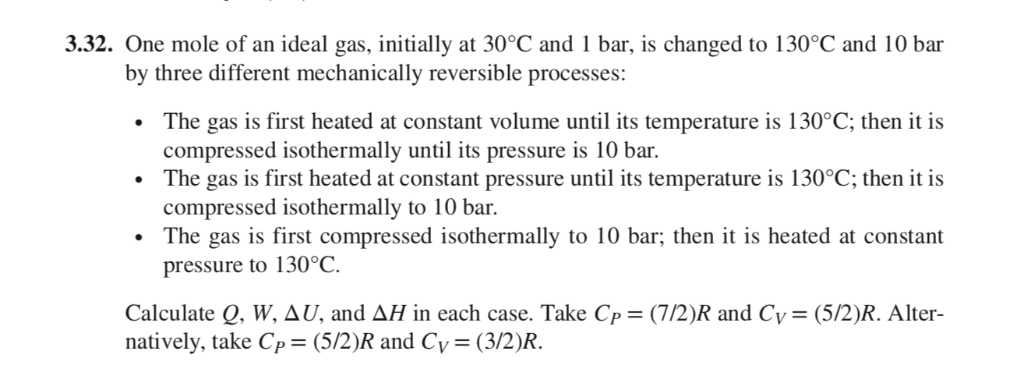3.32. One mole of an ideal gas, initially at 30°C and 1 bar, is changed to 130°C and 10 bar by three different mechanically reversible processes: The gas is first heated at constant volume until its temperature is 130°C; then it is compressed isothermally until its pressure is 10 bar The gas is first heated at constant pressure until its...

• #### answer asap please 2. (25 pts) One mole of a monoatomic ideal gas is initially at state i (P isobanic 150 kPa V-10.0L) and it is being expanded to 2.5 times its original volume along two different pro...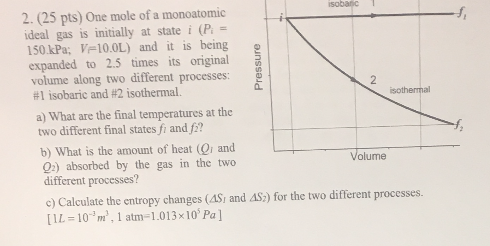answer asap please 2. (25 pts) One mole of a monoatomic ideal gas is initially at state i (P isobanic 150 kPa V-10.0L) and it is being expanded to 2.5 times its original volume along two different processes: £ d. #l isobaric and #2 isothermal. sothermal a) What are the final temperatures at the two different final states fi and...

• #### eBook Video Consider the following data for two variables, z and y. i 135 110 130 145 175 160 120 i 145 100 120 120 130 130 110 a. Consider the four scatter diagrams below. 1. 120 100 80 &#3...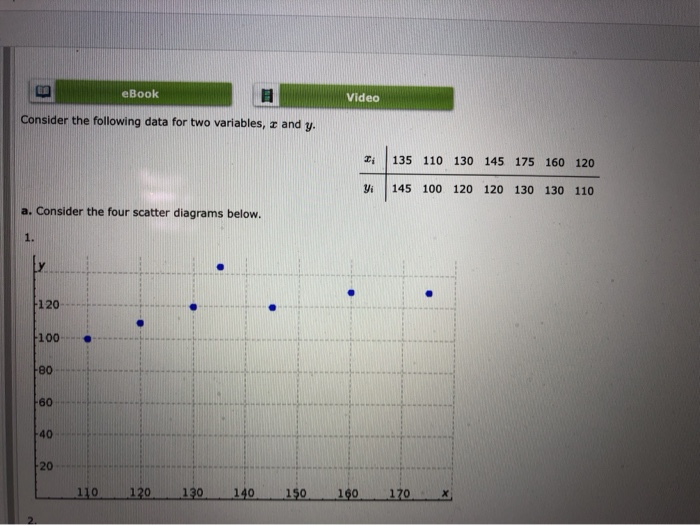eBook Video Consider the following data for two variables, z and y. i 135 110 130 145 175 160 120 i 145 100 120 120 130 130 110 a. Consider the four scatter diagrams below. 1. 120 100 80 " " 60 40 20 110-120 130 149-150 160 170 2. 120 100 80 60 40 20 110-120-130 140 150 160...

• #### Using the Ideal Gas Law, what volume in L would 1 mole of an ideal gas occupy at standard temperature and pressure? Reme...

Using the Ideal Gas Law, what volume in L would 1 mole of an ideal gas occupy at standard temperature and pressure? Remember: STP is 273.15 K and 101.325 kPa.

• #### one mole of an ideal gas initially at a temptuer of Ti=0 undergoes an expansion at a constant...

one mole of an ideal gas initially at a temptuer of Ti=0 undergoes an expansion at a constant perssure of 1.00 atm to four times its original volume. Calculate the newtempterture final of the gas.

• #### One mole of an ideal gas initially at a temperature of Ti = 4°C undergoes an expansion at a...

One mole of an ideal gas initially at a temperature of Ti = 4°C undergoes an expansion at a constant pressure of 1.00 atm, to four times its original volume.

• #### One mole of an ideal monatomic gas initially at 300 K is expanded from an initial pressure of 10 atm to a final pressure of 1 atm

One mole of an ideal monatomic gas initially at 300 K is expanded from an initial pressure of10 atm to a final pressure of 1 atm. Calculate ��E, q, w, ��H, and the final temperature T2 forthis expansion carried out according to each of the following paths. The molar heat capacityat constant volume for a monatomic gas is CV =...

• #### Calculate A, E, μ, cv and S for 1 mole of Kr at 298 K and 1 atm (assuming ideal behavior) Calculate A, E, μ, cv and S for 1 mole of Kr at 298 K and 1 atm (assuming ideal behavior)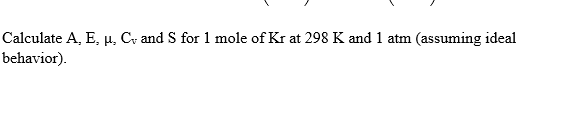Calculate A, E, μ, cv and S for 1 mole of Kr at 298 K and 1 atm (assuming ideal behavior) Calculate A, E, μ, cv and S for 1 mole of Kr at 298 K and 1 atm (assuming ideal behavior)

• #### 2 kg of air, initially at 5 bar, 350 K and 4 Kg of carbon monoxide initially at 2 bar, 450 K are confined to opposite sides of a rigid, well insulated container

2 kg of air, initially at 5 bar, 350 K and 4 Kg of carbon monoxide initially at 2 bar, 450 K are confined to opposite sides of a rigid, well insulated container. The partition is free to move and allows conduction from one gas to the other without energy storage in the partition itself. The air and CO behave...

• #### TB4 The PV diagram in the figure is for n moles of an ideal monatomic gas. The gas is initially at point A. The paths AD and BC represent isothermal changes. R is the universal gas constant. Let the...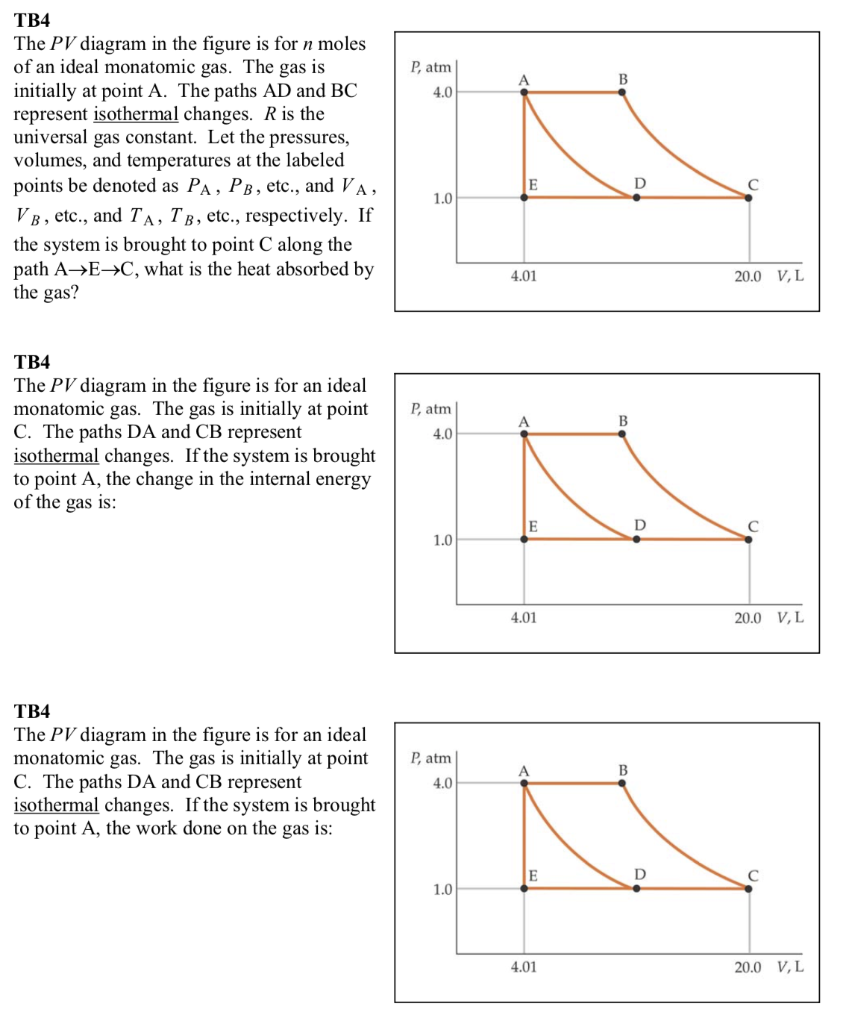TB4 The PV diagram in the figure is for n moles of an ideal monatomic gas. The gas is initially at point A. The paths AD and BC represent isothermal changes. R is the universal gas constant. Let the pressures, volumes, and temperatures at the labeled points be denoted as PA , PB, etc., and VA , VB, etc., and...

• #### For the process CH4(g) = C(s) + 2H2(g) A reaction is started in a rigid vessel at 1000 K with 1 mole of methane and 1 mole of hydrogen gas at 10 bar

For the processCH4(g) = C(s) + 2H2(g)A reaction is started in a rigid vessel at 1000 K with 1 mole of methane and 1 mole of hydrogen gas at 10 bar. Use table C.3 from your text to determine how many grams of carbon should be formed after the reaction has reached equilibrium.

• #### (3). A sample of 1.00 mol ideal gas molecules with Cp, m = 7/2 R is initially at p = 1.00 bar and V = 22.44 L and then p...

(3). A sample of 1.00 mol ideal gas molecules with Cp, m = 7/2 R is initially at p = 1.00 bar and V = 22.44 L and then put thought the following cycle in reversible processes: (a) constant-pressure expansion to twice its initial volume, (b) constant-volume cooling to its initial temperature, (c) isothermal-compression back to 1.00 bar. Calculate q,...

• #### A sample of 1.00 mol ideal gas molecules with Cpm 7/2 R is initially at p 1.00 bar and V 22.44 L and then put tho...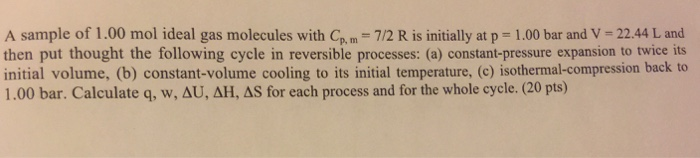A sample of 1.00 mol ideal gas molecules with Cpm 7/2 R is initially at p 1.00 bar and V 22.44 L and then put thought the following cycle in reversible processes: (a) constant-pressure expansion to twice its initial volume, (b) constant-volume cooling to its initial temperature, (c) isothermal-compression back to 1.00 bar. Calculate q, w, AU, AH, AS for...

• #### If an ideal gas initially at 0.6 bar and 1.5 L is compressed at constant temperature to a volume...

If an ideal gas initially at 0.6 bar and 1.5 L is compressed at constant temperature to a volume of 0.8 L, what is the final pressure of the compressed gas

• #### нокти 4. One mole of H2S gas escapes from a container by effusion in 77 seconds. How long would it take one mole of...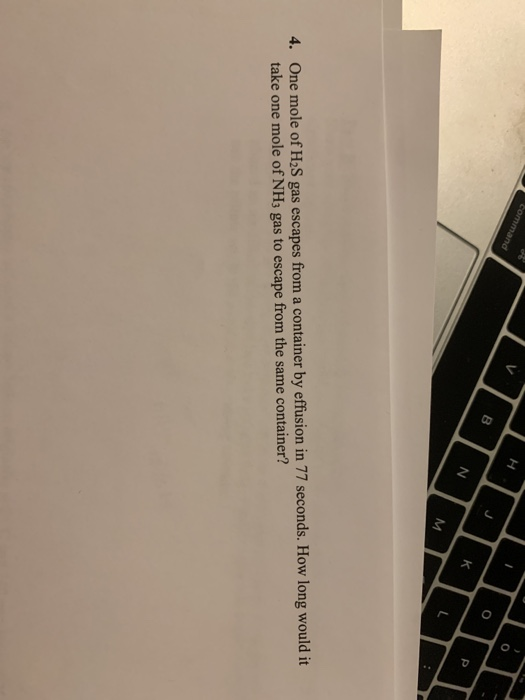нокти 4. One mole of H2S gas escapes from a container by effusion in 77 seconds. How long would it take one mole of NH3 gas to escape from the same container?

• #### Mechanic system u is an mput porce, delermine the stale space b, u reprerentahon op the syilan b2 Mechanic system u is an mput porce, delermine the stale space b, u reprerentahon op the syil...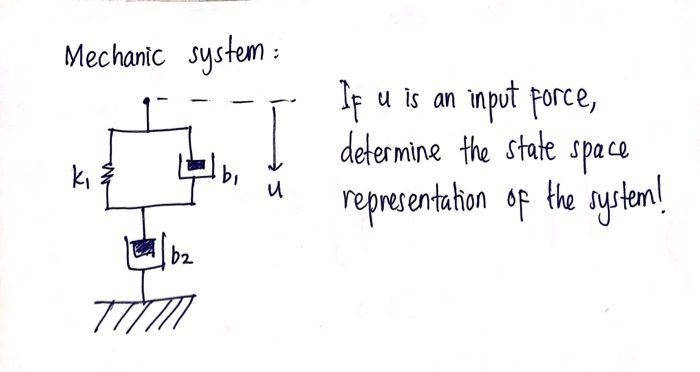Mechanic system u is an mput porce, delermine the stale space b, u reprerentahon op the syilan b2 Mechanic system u is an mput porce, delermine the stale space b, u reprerentahon op the syilan b2

• #### A 20.0-L flask contains 0.400 mole of H2, 0.300 mole of He and 0.500 mole of Ne at 55.0?C. What...

A 20.0-L flask contains 0.400 mole of H2, 0.300 mole of He and 0.500 mole of Ne at 55.0?C. What is the partial pressure of the Ne?

Need Online Homework Help?